Question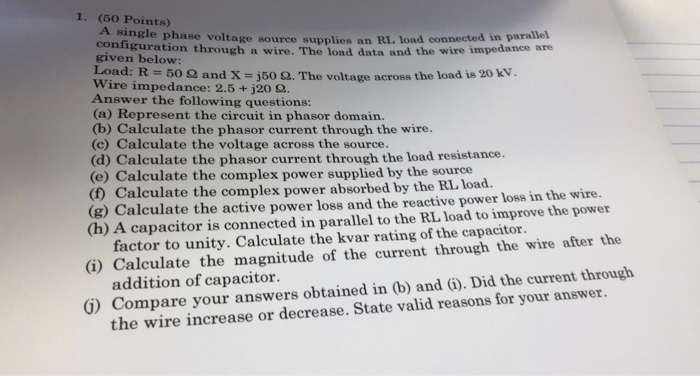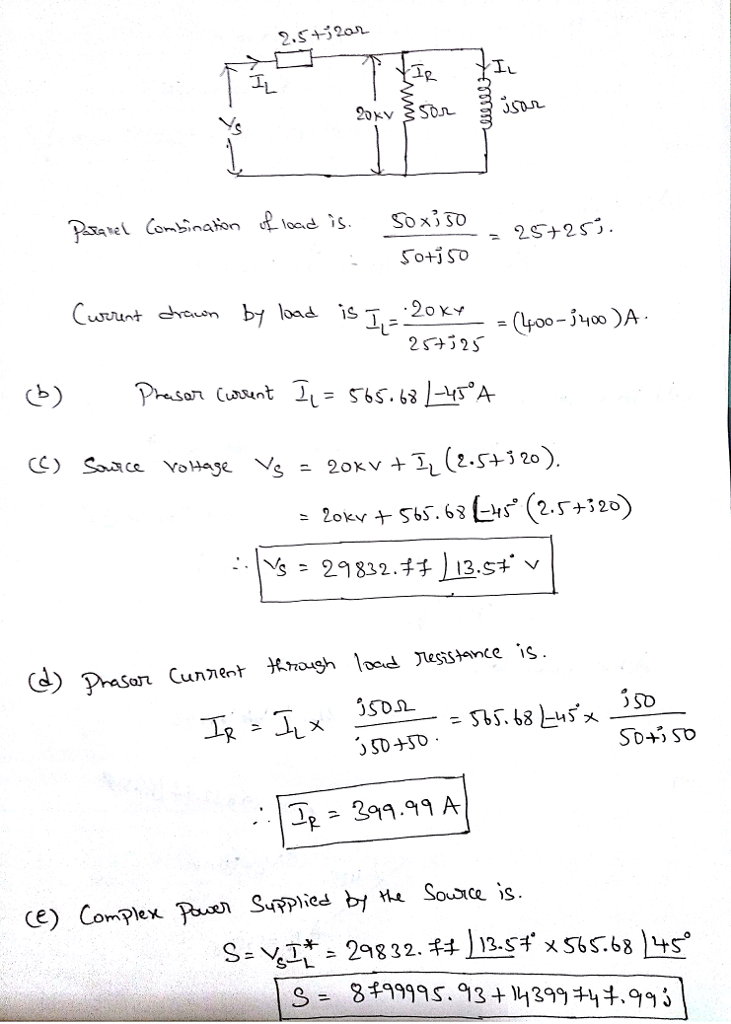#### Earn Coins

Coins can be redeemed for fabulous gifts.

Similar Homework Help Questions
• ### 50 Points 1- A balanced delta connected load of 15 + j18 per phase is connected...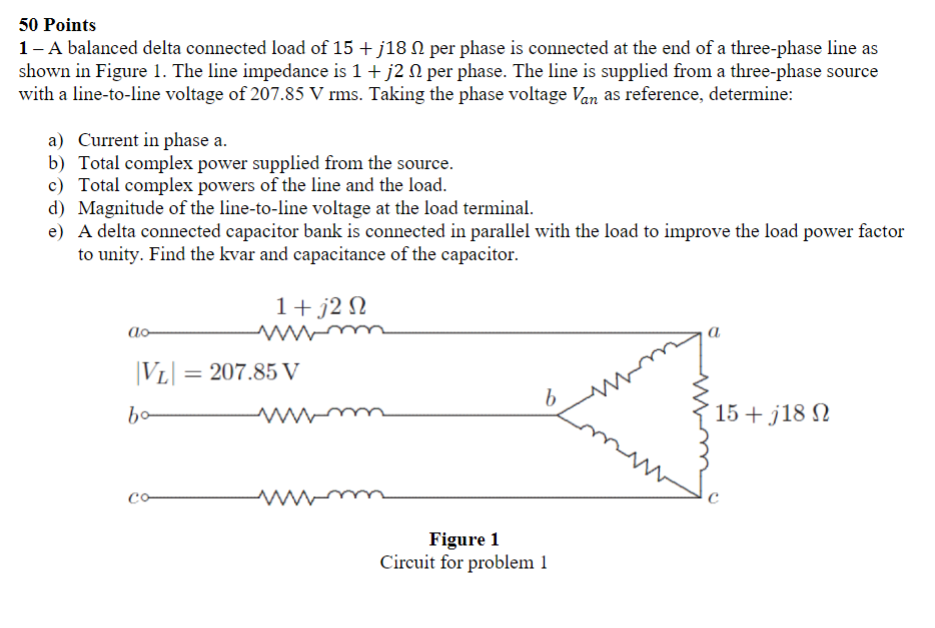50 Points 1- A balanced delta connected load of 15 + j18 per phase is connected at the end of a three-phase line as shown in Figure 1. The line impedance is 1 + 12 12 per phase. The line is supplied from a three-phase source with a line-to-line voltage of 207.85 V rms. Taking the phase voltage Van as reference, determine: a) Current in phase a. b) Total complex power supplied from the source. c) Total complex powers of...

• ### 1- A balanced delta connected load of 15 + j18 12 per phase is connected at...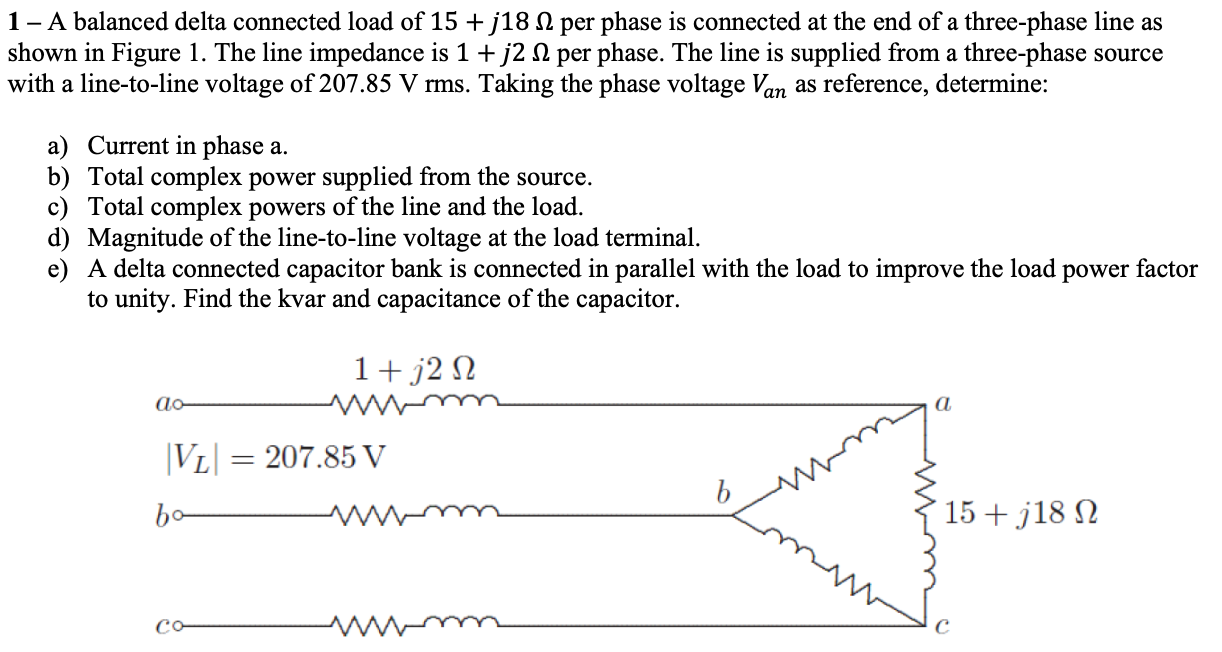1- A balanced delta connected load of 15 + j18 12 per phase is connected at the end of a three-phase line as shown in Figure 1. The line impedance is 1 + j2 12 per phase. The line is supplied from a three-phase source with a line-to-line voltage of 207.85 V rms. Taking the phase voltage Van as reference, determine: a) Current in phase a. b) Total complex power supplied from the source. c) Total complex powers of the...

• ### A balanced delta connected load of 15+?18 Ω per phase is connected at the end of...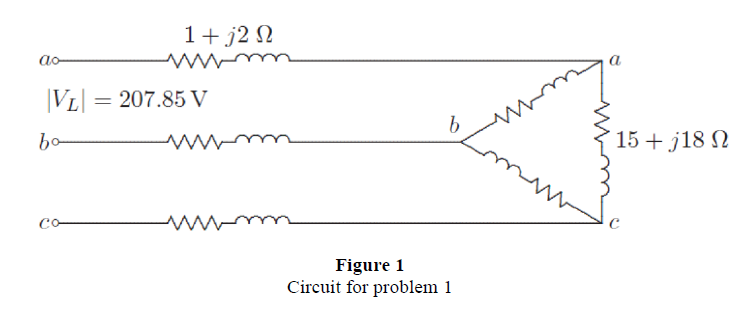A balanced delta connected load of 15+?18 Ω per phase is connected at the end of a three-phase line as shown in Figure 1. The line impedance is 1+?2 Ω per phase. The line is supplied from a three-phase source with a line-to-line voltage of 207.85 V rms. Taking the phase voltage ??? as reference, determine: 1. Total complex powers of the line and the load. 2.A delta connected capacitor bank is connected in parallel with the load to improve...

• ### 5. The line to neutral voltages of a Y-connected three phase source are given by, The...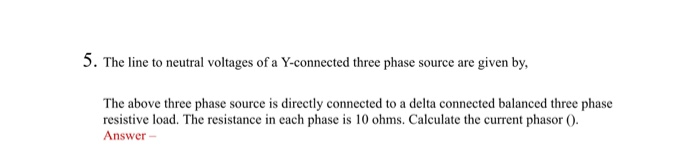5. The line to neutral voltages of a Y-connected three phase source are given by, The above three phase source is directly connected to a delta connected balanced three phase resistive load. The resistance in each phase is 10 ohms. Calculate the current phasor 0. Answer- 4. Following three loads are connected in parallel across a single-phase source voltage source: Load 1 absorbs 15 kW and 6.667 kvar; Load 2 absorbs 3 kVA at 0.96PF leading; Load 3 absorbs 15...

• ### Series and parallel RL and RC circuits with an AC source. A 60 Hz voltage source...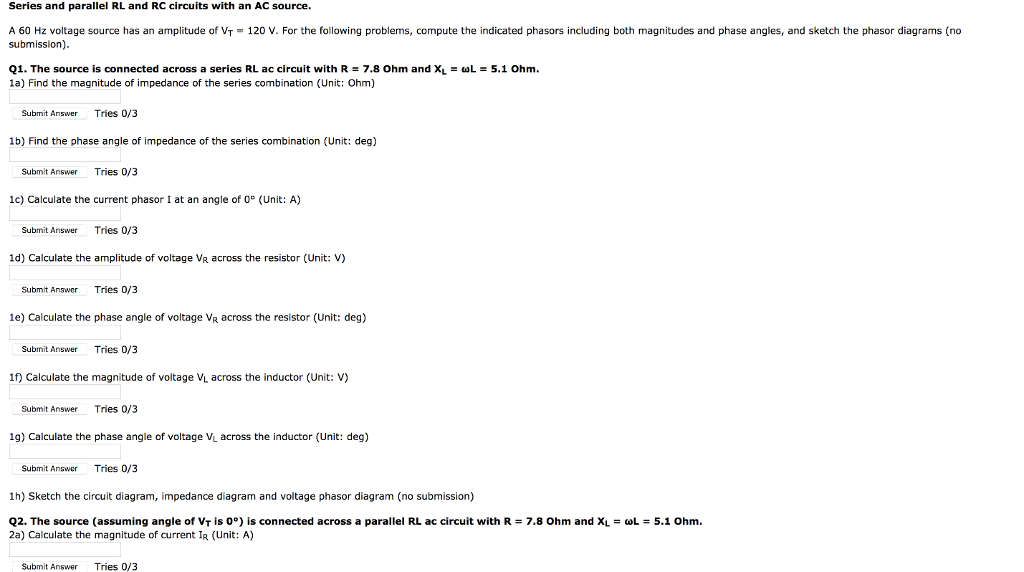Series and parallel RL and RC circuits with an AC source. A 60 Hz voltage source has an amplitude of VT-120 V. For the following problems, compute the indicated phasors including both magnitudes and phase angles, and sketch the phasor diagrams (no submission) Q1. The source is connected across a series RL ac circuit with R 7.8 Ohm and XL = ωし= 5.1 Ohm. 1a) Find the magnitude of impedance of the series combination (Unit: Ohm) Submit Answer Tries 0/3...

• ### A three-phase load of 15 kVA with a PF of 0.8 lagging is connected in parallel...

A three-phase load of 15 kVA with a PF of 0.8 lagging is connected in parallel with a three-phase load of 36 kW at 0.6 PF leading. The line-line voltage is 2000V. a) Find the total complex power and power factor. Is it inductive or capacitive? b) How much kVAR is needed to make the PF unity? c) What is the magnitude of the current going into the total load?

• ### 1. A resistor R and capacitor care connected in series with an AC voltage source with...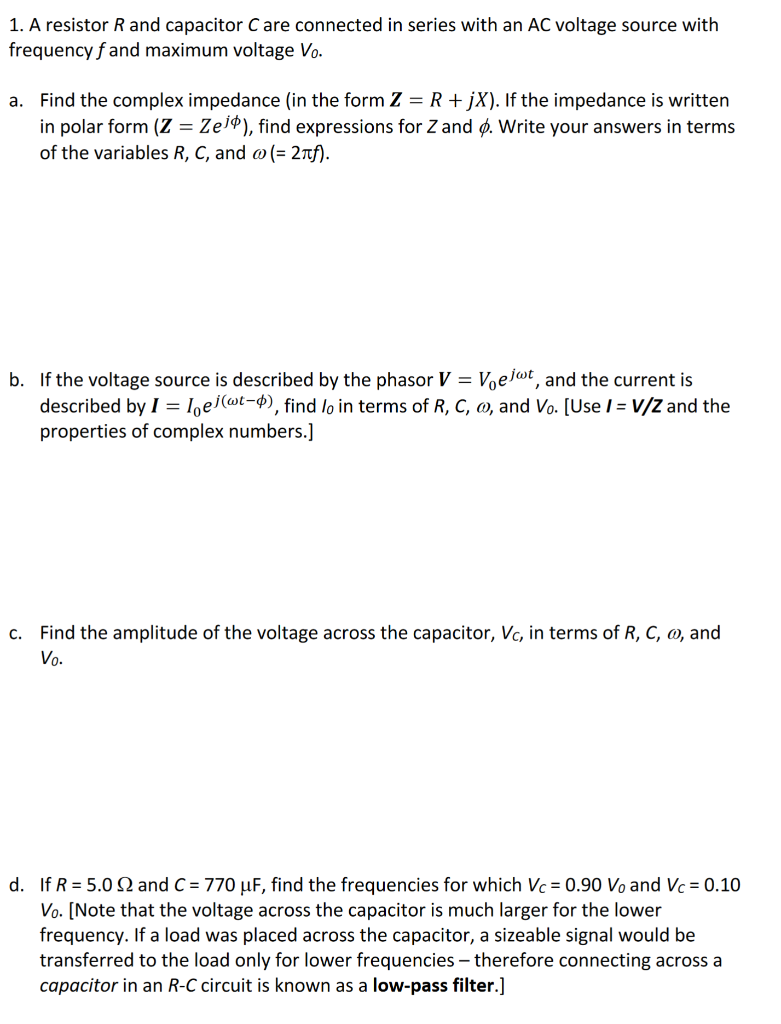1. A resistor R and capacitor care connected in series with an AC voltage source with frequency f and maximum voltage Vo. a. Find the complex impedance (in the form Z = R + jX). If the impedance is written in polar form (Z = Zejº), find expressions for Z and Ⓡ. Write your answers in terms of the variables R, C, and o(= 21f). b. If the voltage source is described by the phasor V = V, ejwt, and...

• ### 1. A 480 V (rms) source supplies power to two loads connected in parallel. The first...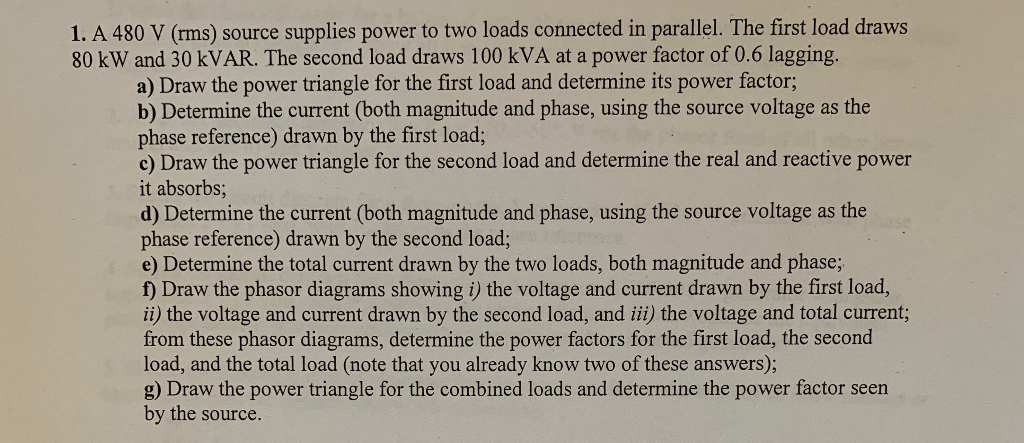1. A 480 V (rms) source supplies power to two loads connected in parallel. The first load draws 80 kW and 30 kVAR. The second load draws 100 kVA at a power factor of 0.6 lagging. a) Draw the power triangle for the first load and determine its power factor; b) Determine the current (both magnitude and phase, using the source voltage as the phase reference) drawn by the first load; c) Draw the power triangle for the second load...

• ### A balanced Y-connected voltage source with a phase voltage ?௔௡ = 277∠0°? delivers to a balanced...

A balanced Y-connected voltage source with a phase voltage ?௔௡ = 277∠0°? delivers to a balanced delta connected load with impedance ?ௗ௘௟௧௔ = 45 − ?18Ω that is in parallel with a Yconnected load with impedance ?௒ = 30 + ?15Ω. (a) Calculate the current at the source (b) Calculate the source real and reactive power

• ### A Δ-connected source supplies power to a Y-connected load in a three-phase balanced system. Given that...

A Δ-connected source supplies power to a Y-connected load in a three-phase balanced system. Given that the line impedance is (1 + j1) Ω per phase while the load impedance is (6 + j4) Ω per phase, find the magnitude of the line voltage at the load. Assume the source phase voltage Vab= 208 ∠0° V rms. The magnitude of the line voltage at the load is _______ Vrms.